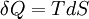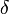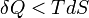# Heat$\delta Q= TdS$
where T is the temperature and S is the entropy.$\delta$ represents an inexact differential, indicating that the resulting value depends on the path taken. This equation is an equality when the process is reversible, i.e., the system evolves through a succession of equilibrium states. If the process is irreversible one has$\delta Q < TdS$